# Short Strangle Payoff and Break-Even Points

This page explains short strangle profit and loss at expiration and the calculation of its break-even points.

## Short Strangle Basic Characteristics

Short strangle is a position created by selling a higher strike call option and selling a lower strike put option with the same expiration date.

It is a non-directional short volatility strategy, typically used when a trader expects the underlying price to not move much during the time until expiration, or more generally, expects future realized volatility to be smaller than implied volatility priced in the options.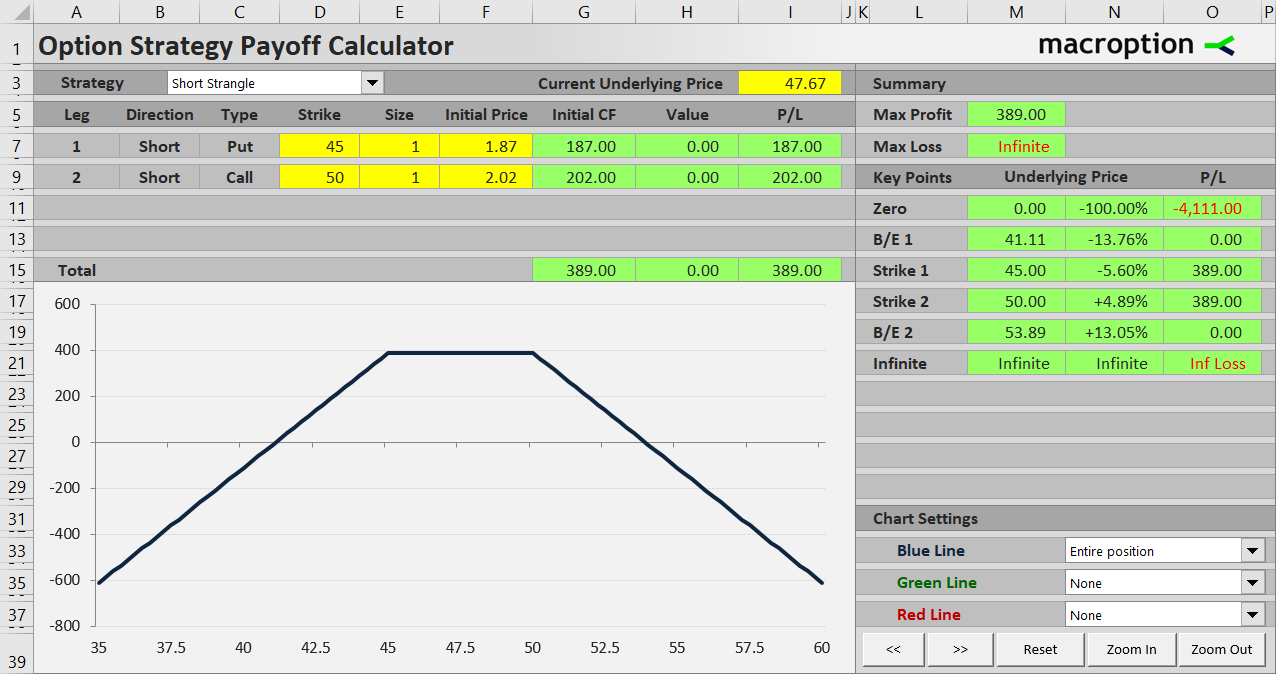Short strangle is inverse to long strangle and similar to short straddle. It has limited profit potential and unlimited risk. Therefore a short strangle position must be carefully managed, otherwise it can cause huge losses when volatility explodes.

We will use an example to illustrate the payoff profile and explain the calculation of maximum profit and break-even points. If you have seen the long strangle payoff tutorial, you will notice that we are using the exact opposite position – the other side of the trade from our long strangle example.

## Short Strangle Example

We will create a short strangle position by doing the following:

Sell a \$50 strike call option for \$2.02 per share.

Sell a \$45 strike put option with the same expiration date for \$1.87 per share.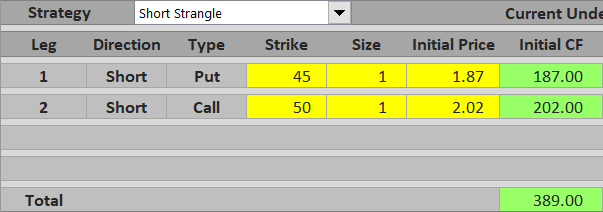In our example:

Premium received = \$2.02 + \$1.87 = \$3.89 per share = \$389 for one option contract

## Maximum Possible Profit

The premium received is actually the most we can make from the entire trade. Because we are short both the options, there is no way to make additional gains from them – at best both the options will expire worthless and we keep the entire premium.

Maximum profit from a short strangle trade equals premium received. It applies when underlying price ends up between the two strikes at expiration, or is exactly equal to one of the strikes.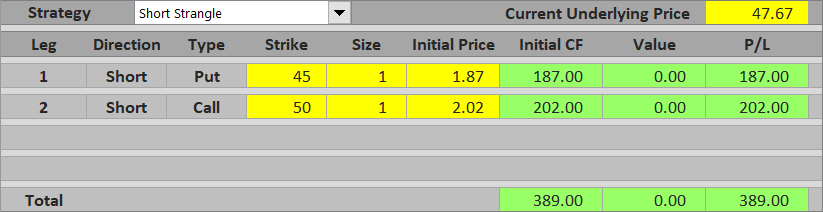If we are wrong with our low volatility expectations and underlying price does make a big move in one or the other direction, we may lose the entire premium received and possibly much more. It is therefore very important to understand what happens when underlying price moves beyond one or the other strike.

## Underlying Above Call Strike

If underlying price climbs above the call option strike, this option gets in the money. Because we are short, any increase in the option's value reduces our profit or increases our loss. On the other hand, the put option is out of the money under this scenario and has no further effect on the trade's P/L.

For example, if underlying price ends up at \$51.50 at expiration, the call option is in the money by \$51.50 – \$50 = \$1.50 per share. We will most likely be assigned at expiration and lose \$150. The put option has no effect. Because we have received \$389 for both options in the beginning, our total P/L from this trade is \$389 – \$150 = \$239. The underlying price going above the call strike has reduced our profit, but fortunately it didn't get high enough to turn the trade into a loss.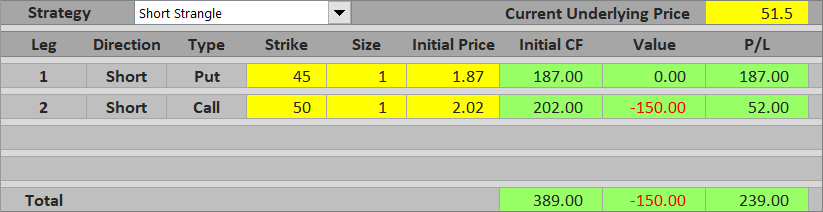If it did move higher, let's say to \$58, the short call option's value would be \$800 and high enough to exceed the premium received. The overall result would be a loss: \$389 – \$800 = – \$411.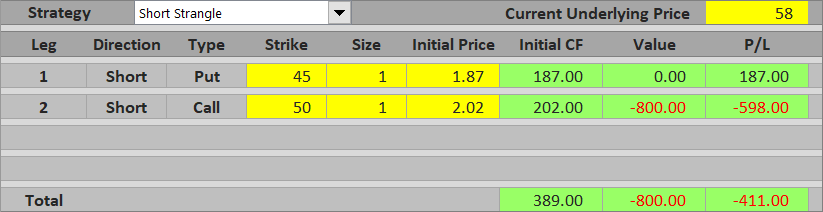Theoretically, at least for most securities, there is no limit on how high the underlying price can go and therefore no limit on the potential loss from a short strangle trade.

## Underlying Below Put Strike

If underlying price makes a big move to the downside and falls below the put option's strike, the put will get in the money. The logic is the same as above, only the roles of the two options have switched. The call is now out of the money and has no further effect on P/L. If underlying price gets below the put strike by just a small amount, the trade will still end with profit, although lower than premium received.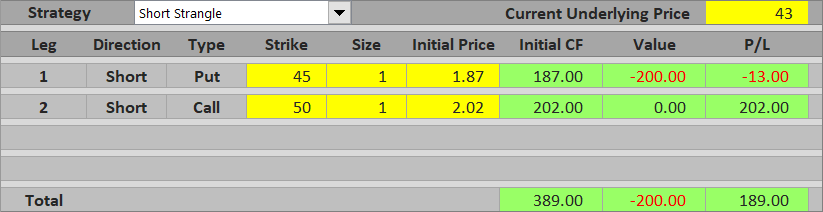If underlying price drops by a larger amount, our short strangle makes a loss.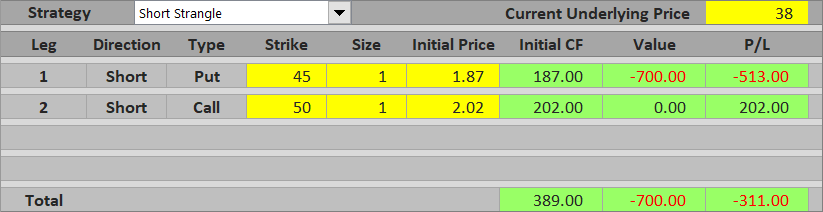Unlike to the upside, the losses are not unlimited, because the worst case scenario is the underlying price falling to zero (at least with most underlying securities). That said, typically the loss in such case is so high that it is almost like unlimited. It can actually be calculated quite easily:

Maximum loss if underlying price goes down = put strike – premium received

... where premium received is from both the call and the put, not just the put.

In our example it is \$45 – \$3.89 = \$41.11 per share = \$4,111 for one contract.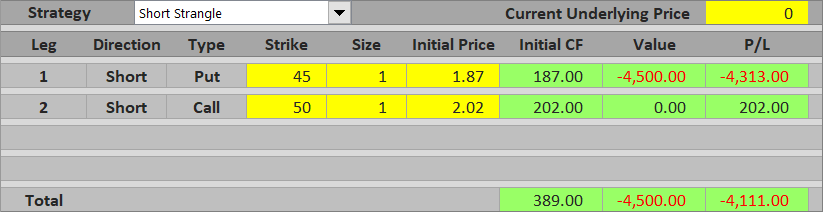## Short Strangle Break-Even Points

The exact underlying price where an option strategy's payoff turns from profit to loss or vice versa is known as break-even point. Short strangle has two such points – one below the put strike and one above the call strike.

The calculation of short strangle break-even points is the same as for long strangle, which is not that surprising as these two strategies are the inverse of one another. The formulas are:

B/E #1 = put strike – initial cost

B/E #2 = call strike + initial cost

You can also see than the first break-even point is the same value as the maximum loss when underlying price falls to zero, which we have calculated above.

In our example the break-evens are:

B/E #1 = \$45 – \$3.89 = \$41.11

B/E #2 = \$50 + \$3.89 = \$53.89

## Short Strangle Payoff Summary

Below you can find a short strangle payoff diagram (blue) with contributions of the two legs – the short put (green) and the short call (red).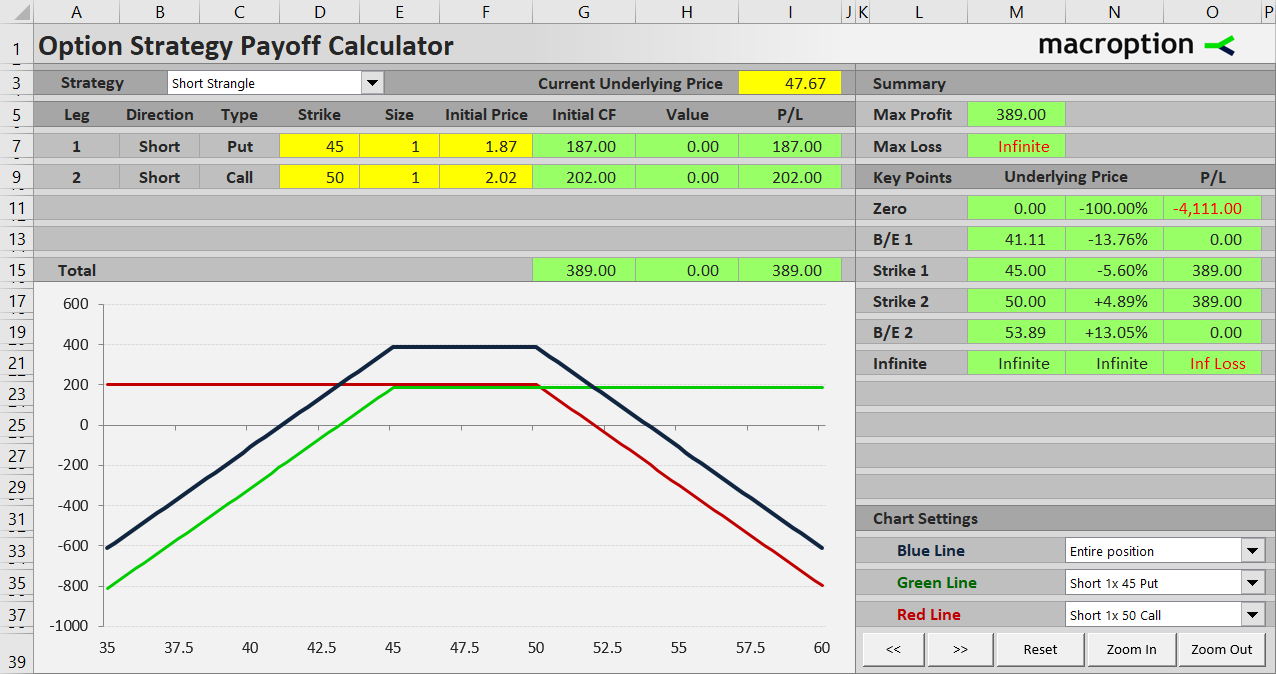If underlying price stays between the two strikes (\$45 and \$50), or is exactly equal to one of them at expiration, the trade makes maximum profit equal to premium received from selling both the options.

Above the call strike (\$50) total profit decreases as underlying price rises. At the break-even point (\$53.89), equal to call strike + premium received, the trade turns into a loss.

Similarly, below the put strike (\$45) total profit decreases proportionally to underlying price. At the break-even point (\$41.11), equal to put strike – premium received, the trade turns into a loss.

Maximum possible loss is very high to the downside and unlimited to the upside.

## Similar Option Strategies

We already know that short strangle is the other side of long strangle.

It is very similar to short straddle, which is the other side of long straddle. The difference between straddle and strangle is that in a straddle the call and the put have the same strike price, while in a strangle the call strike is higher than the put strike.

Short strangle is also similar to short guts, which is the other side of long guts. With these strategies the put strike is higher than the call strike, so both the options are typically in the money when opening the position.

Not least, short strangle is closely related to iron condor, which is actually a short strangle hedged with a long strangle with wider gap between the strikes. This reduces premium received and thereby profit potential, but it also significantly limits the risk of the trade.

By remaining on this website or using its content, you confirm that you have read and agree with the Terms of Use Agreement.

We are not liable for any damages resulting from using this website. Any information may be inaccurate or incomplete. See full Limitation of Liability.

Content may include affiliate links, which means we may earn commission if you buy on the linked website. See full Affiliate and Referral Disclosure.

We use cookies and similar technology to improve user experience and analyze traffic. See full Cookie Policy.